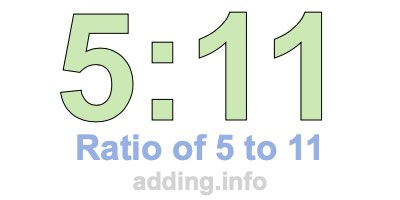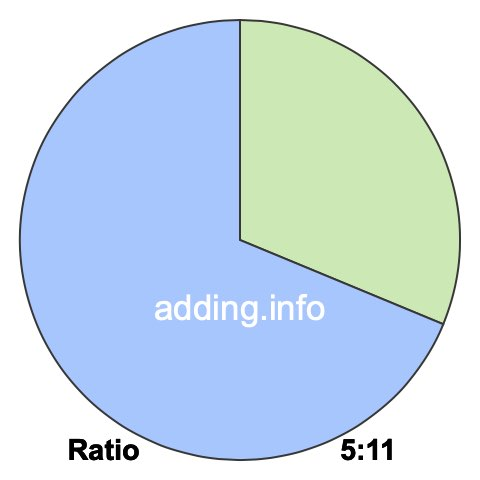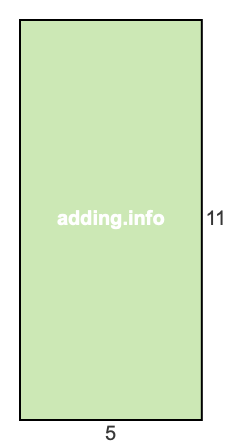Ratio of 5 to 11A ratio of 5 to 11 can be written as 5 to 11, 5:11, or 5/11. Furthermore, 5 and 11 can be the quantity or measurement of anything, such as students, fruit, weights, heights, speed and so on.

A ratio of 5 to 11 simply means that for every 5 of something, there are 11 of something else, with a total of 16.

In the box below, you can enter a number in either box and we will calculate the other number to keep the ratio of 5 to 11. In other words, we will calculate the corresponding equivalent ratio of 5:11 for you.

:

To calculate the left side of the ratio, our calculator multiplies the right side by 5 and then divides the product by 11. To calculate the right side of the ratio, our calculator multiplies the left side by 11 and then divides the product by 5. Answers are rounded to two decimals if necessary.

Below is a list of equivalent ratios of 5:11 that we created using our 5:11 ratio calculator above.

5:11

10:22

15:33

20:44

25:55

30:66

35:77

40:88

45:99

50:110

55:121

60:132

We have also created a pie chart for you to put 5:11 ratio in perspective. To do so, we divided a pie into 16 pieces and then colored 5 pieces green and 11 pieces blue. The pie will look exactly the same using any equivalent ratio of 5:11.The green part of the pie covers 31.25% and the blue part covers 68.75%. To calculate the percentages, we divided each side of the ratio by its total and then multiplied by 100, like this:

(5 / 16)×100 = 31.25
(11 / 16)×100 = 68.75

In fractional terms, 5/11 of the pie above is colored in green. This means that the ratio of 5 to 11 or 5:11 can also be expressed as a fraction, where the 5 to the left of the colon is the numerator and the 11 to the right of the colon is the denominator:

5:11 = 5/11

Ratio is often used when defining rectangles in width:height format. Width and height can be feet, inches, centimeters, or any other kind of length. With a ratio of 5 to 11, the width is 5 and the height is 11. Below is a picture of what a rectangle with a ratio of 5:11 looks like.Since the simplest form of the fraction 5/11 is 5/11, the simplest form of the ratio 5:11 is also 5:11.

Ratio Calculator
Need information about another ratio? Here you can submit another ratio for us to explain.

:

Ratio of 5 to 12
Go to the next ratio on our list that we have detailed information about.